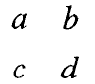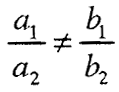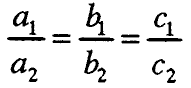# System of linear equations and inequalities

### 1. SYSTEM LINEAR EQUATIONS WITH TWO VARIABLES

The conjunction of two equations f (x, y) = 0 and g (x, y) = 0 with two variables, ie.
f (x, y) = 0 ∧ g (x, y) = 0 or

is called a system of equations with two variables.

If MZ and MZ are the sets of solutions of the equations f (x, y) = 0 and g (x, y) = 0, then MZ ∩ MZ is the set of solutions of the system of equations. Any system of 2 linear equations with two variables can be generalized:

where x and y are variables and a₁, a₂, b₁, b₂, c₁, care any real numbers or expressions that do not depend on the variables.

The numbers a₁, a₂, b₁, b₂ are called coefficients before the variables, and c₁, c₂ – free members of the system.

#### 1.1. Solving a system of linear equations with two variables

1 °. For two systems of equations, we say that they are equivalent if they have equal sets of solutions.
2 °. If any of the equations of a given system is replaced by an equivalent equation, a system equivalent to the given one is obtained.
3 °. If from an equation of the system

one variable (e.g. u) can be uniquely expressed through the other variable: у = g (x), then the system of equations

is equivalent to the first, ie. (1) <=> (2).

The substitution method for solving a system of two equations with two variables is based on this claim.
4 °. The system of equations:

is equivalent to the system of equations:

where k is any real number. This method is based on the method of opposite coefficients for solving a system of two equations with two variables.

#### 1.2. Second order determinants and their application

The quadratic pattern joined by the number a d – b c is called the second order determinant. Enroll:

The numbers a and d make up the main diagonal, and the numbers c and b – the side diagonal of the determinant.

So, the value of the second order determinant is equal to the difference of the products of its elements from the main and the secondary diagonal.

The system of equations

provided that a₁ b₂ – a₂ b₁ ≠ 0 has a unique solution (2):

If the expressions in formula (2) are denoted by the determinants:

then the components of the system solution (1) can be written as follows:

at ≠ ≠ 0, the formulas are called Kramer formulas.

#### 1.3. Discussion of solutions of a system of linear equations with two variables

Discuss the solutions of the system of linear equations with two variables

means to determine in which relations between the coefficients (assuming they are different from zero), he has a single solution, there is no solution or there are infinitely many solutions.

1 °. If,then the system is solvable, ie. there is only one solution.

2 °. If,then the system has no solution, ie. he is unsolvable.

3 °. If so, then the system is solvable and has infinitely many solutions.

#### 1.4. Graphical solution system of linear equations with two variables

1) The system of linear equations with two variables has a unique solution if the graphs of the equations of the system – the lines р₁ and р₂ intersect. The coordinates of the intersection point Ѕ, ie. Ѕ (x₀, UZ) is a solution of the system of equations.

Fig. 1

2) The system of linear equations with two variables has no solution, if the graphs of the equations of the system – lines рand р₂ do not have a common point, ie when the lines are different parallel lines (fig. 2)

(Fig. 2).

3) The system of linear equations with two variables has infinitely many solutions if the graphs of the equations of the system – the lines р₁ and р₂ are parallel lines that match

(Fig. 3).

### 2. SYSTEMS OF THREE LINEAR EQUATIONS WITH THREE VARIABLES

The conjugation of three linear equations

is called a system of three linear equations with three unknowns.

The ordered triple of numbers (x₀, y₀, z₀) for which the conjunction is a true statement is a solution of the system of equations.

Any system of three linear equations with three variables can be generalized:

where x, y, z are variables.

The numbers ai, bi, si (i = 1,2,3) are called coefficients before the variables, and d1 (i = 1,2,3) – free members of the system.

#### 2.1. METHODS FOR SOLVING A SYSTEM OF THREE LINEAR EQUATIONS WITH THREE VARIABLES

##### 1) Replacement method

We solve one of the equations of the system by any variable and with the expression found for it, we replace the corresponding variable in the other two equations of the system, thus obtaining a system of two equations with two variables. After solving the resulting system, we replace the values for both variables in the expression of the third variable. Thus, one solution of the system is determined.

##### 2) Elimination method

We will show this method in the following example.
Example.

##### 3) Solve a system of three linear equations with three variables with third order determinants

The square pattern that the number joins

is called the third order determinant, ie.

The value of the determinant can be calculated more easily and quickly by applying the so-called Sarus rule, given by the following scheme:

Let the system of equations be given:

We can form the following determinants:

If ∆ ≠ 0, then to solve the system of equations (1) we use the formulas:

called Kramer formulas. The subordinate trio

is a solution of the system (1).

### 3. SYSTEM LINEAR INEQUALITIES WITH ONE UNKNOWN

The conjunction of two inequalities A <B and C <D with one unknown, ie.

(A <B) ∧ (C <D) or iscalled a system of two inequalities with one unknown.

Example. Let's solve the system of inequalities

We reduce the system to the equivalent system:

The intersection of the sets of solutions of each inequality separately, ie.

M = M₁ ∩ M₂

is a set of system solutions:

M = M₁ ∩ M₂ = (-∞, – 3/2) ∩ (-3, + ∞) = (-3, – 3/2)
Graphically, the system solution is shown in Figure 1.

Fig. 1

### 4. GRAPHIC SOLUTION OF A SYSTEM OF LINEAR INEQUALITIES WITH TWO VARIABLES

A system of linear inequalities with two variables means a conjunction of two or more linear inequalities with two variables. The set of solutions of the system of linear inequalities is the intersection of the sets of solutions of the inequalities that enter the system. Thus, if the resulting set is not empty, then the system is solvable, and if the intersection is an empty set – the system is unsolvable.

Example. Let's solve the graphical system of inequalities:

2х-у + 4> 0 ∧ х + у + 2> 0

Fig. 2

The double-hatched part is the solution to the system of inequalities.

###### – system of linear equations and non-equations –
Total Page Visits: 466 - Today Page Visits: 2